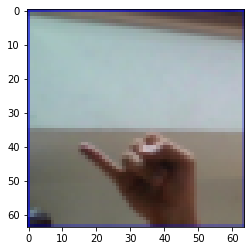Learn data science and machine learning by building real-world projects on Jovian

## Sign Language Detection - An Image Classification Project

The data set is a collection of images of alphabets from the American Sign Language, separated in 29 folders which represent the various classes.

#### Content

The training data set contains 87,000 images which are 200x200 pixels. There are 29 classes, of which 26 are for the letters A-Z and 3 classes for SPACE, DELETE and NOTHING. These 3 classes are very helpful in real-time applications, and classification. The test data set contains a mere 28 images, to encourage the use of real-world test images.

### Importing important libraries and project creation.

In :
``````import os
import torch
import torchvision
import tarfile
import torch.nn as nn
import numpy as np
import torch.nn.functional as F
from torchvision.datasets import ImageFolder
import torchvision.transforms as tt
from torch.utils.data import random_split
from torchvision.utils import make_grid
import matplotlib
import matplotlib.pyplot as plt
%matplotlib inline

matplotlib.rcParams['figure.facecolor'] = '#ffffff'``````
In :
``project_name='sign-detection'``

### Retrieving sign language dataset from Kaggle datasets.

In :
``````from google.colab import drive
drive.mount('/content/gdrive')``````
```Drive already mounted at /content/gdrive; to attempt to forcibly remount, call drive.mount("/content/gdrive", force_remount=True). ```
In :
``````import os
os.environ['KAGGLE_CONFIG_DIR'] = "/content/gdrive/My Drive/Kaggle"``````
In :
``%cd /content/gdrive/My Drive/Kaggle``
```/content/gdrive/My Drive/Kaggle ```
In :
``!kaggle datasets download -d grassknoted/asl-alphabet``
```asl-alphabet.zip: Skipping, found more recently modified local copy (use --force to force download) ```
In :
``!unzip \*.zip  && rm *.zip``
```Archive: asl-alphabet.zip replace asl_alphabet_test/asl_alphabet_test/A_test.jpg? [y]es, [n]o, [A]ll, [N]one, [r]ename: n replace asl_alphabet_test/asl_alphabet_test/B_test.jpg? [y]es, [n]o, [A]ll, [N]one, [r]ename: n replace asl_alphabet_test/asl_alphabet_test/C_test.jpg? [y]es, [n]o, [A]ll, [N]one, [r]ename: n replace asl_alphabet_test/asl_alphabet_test/D_test.jpg? [y]es, [n]o, [A]ll, [N]one, [r]ename: n replace asl_alphabet_test/asl_alphabet_test/E_test.jpg? [y]es, [n]o, [A]ll, [N]one, [r]ename: n replace asl_alphabet_test/asl_alphabet_test/F_test.jpg? [y]es, [n]o, [A]ll, [N]one, [r]ename: n replace asl_alphabet_test/asl_alphabet_test/G_test.jpg? [y]es, [n]o, [A]ll, [N]one, [r]ename: n replace asl_alphabet_test/asl_alphabet_test/H_test.jpg? [y]es, [n]o, [A]ll, [N]one, [r]ename: n replace asl_alphabet_test/asl_alphabet_test/I_test.jpg? [y]es, [n]o, [A]ll, [N]one, [r]ename: n n replace asl_alphabet_test/asl_alphabet_test/J_test.jpg? [y]es, [n]o, [A]ll, [N]one, [r]ename: replace asl_alphabet_test/asl_alphabet_test/K_test.jpg? [y]es, [n]o, [A]ll, [N]one, [r]ename: n replace asl_alphabet_test/asl_alphabet_test/L_test.jpg? [y]es, [n]o, [A]ll, [N]one, [r]ename: n n replace asl_alphabet_test/asl_alphabet_test/M_test.jpg? [y]es, [n]o, [A]ll, [N]one, [r]ename: replace asl_alphabet_test/asl_alphabet_test/N_test.jpg? [y]es, [n]o, [A]ll, [N]one, [r]ename: n replace asl_alphabet_test/asl_alphabet_test/O_test.jpg? [y]es, [n]o, [A]ll, [N]one, [r]ename: n replace asl_alphabet_test/asl_alphabet_test/P_test.jpg? [y]es, [n]o, [A]ll, [N]one, [r]ename: n replace asl_alphabet_test/asl_alphabet_test/Q_test.jpg? [y]es, [n]o, [A]ll, [N]one, [r]ename: n replace asl_alphabet_test/asl_alphabet_test/R_test.jpg? [y]es, [n]o, [A]ll, [N]one, [r]ename: n replace asl_alphabet_test/asl_alphabet_test/S_test.jpg? [y]es, [n]o, [A]ll, [N]one, [r]ename: n replace asl_alphabet_test/asl_alphabet_test/T_test.jpg? [y]es, [n]o, [A]ll, [N]one, [r]ename: n replace asl_alphabet_test/asl_alphabet_test/U_test.jpg? [y]es, [n]o, [A]ll, [N]one, [r]ename: n replace asl_alphabet_test/asl_alphabet_test/V_test.jpg? [y]es, [n]o, [A]ll, [N]one, [r]ename: n n replace asl_alphabet_test/asl_alphabet_test/W_test.jpg? [y]es, [n]o, [A]ll, [N]one, [r]ename: replace asl_alphabet_test/asl_alphabet_test/X_test.jpg? [y]es, [n]o, [A]ll, [N]one, [r]ename: n replace asl_alphabet_test/asl_alphabet_test/Y_test.jpg? [y]es, [n]o, [A]ll, [N]one, [r]ename: n replace asl_alphabet_test/asl_alphabet_test/Z_test.jpg? [y]es, [n]o, [A]ll, [N]one, [r]ename: n replace asl_alphabet_test/asl_alphabet_test/nothing_test.jpg? [y]es, [n]o, [A]ll, [N]one, [r]ename: n replace asl_alphabet_test/asl_alphabet_test/space_test.jpg? [y]es, [n]o, [A]ll, [N]one, [r]ename: n replace asl_alphabet_train/asl_alphabet_train/A/A1.jpg? [y]es, [n]o, [A]ll, [N]one, [r]ename: n replace asl_alphabet_train/asl_alphabet_train/A/A10.jpg? [y]es, [n]o, [A]ll, [N]one, [r]ename: n replace asl_alphabet_train/asl_alphabet_train/A/A100.jpg? [y]es, [n]o, [A]ll, [N]one, [r]ename: n replace asl_alphabet_train/asl_alphabet_train/A/A1000.jpg? [y]es, [n]o, [A]ll, [N]one, [r]ename: n replace asl_alphabet_train/asl_alphabet_train/A/A1001.jpg? [y]es, [n]o, [A]ll, [N]one, [r]ename: n replace asl_alphabet_train/asl_alphabet_train/A/A1002.jpg? [y]es, [n]o, [A]ll, [N]one, [r]ename: n replace asl_alphabet_train/asl_alphabet_train/A/A1003.jpg? [y]es, [n]o, [A]ll, [N]one, [r]ename: n n replace asl_alphabet_train/asl_alphabet_train/A/A1004.jpg? [y]es, [n]o, [A]ll, [N]one, [r]ename: replace asl_alphabet_train/asl_alphabet_train/A/A1005.jpg? [y]es, [n]o, [A]ll, [N]one, [r]ename: n replace asl_alphabet_train/asl_alphabet_train/A/A1006.jpg? [y]es, [n]o, [A]ll, [N]one, [r]ename: n replace asl_alphabet_train/asl_alphabet_train/A/A1007.jpg? [y]es, [n]o, [A]ll, [N]one, [r]ename: n replace asl_alphabet_train/asl_alphabet_train/A/A1008.jpg? [y]es, [n]o, [A]ll, [N]one, [r]ename: n replace asl_alphabet_train/asl_alphabet_train/A/A1009.jpg? [y]es, [n]o, [A]ll, [N]one, [r]ename: n replace asl_alphabet_train/asl_alphabet_train/A/A101.jpg? [y]es, [n]o, [A]ll, [N]one, [r]ename: n replace asl_alphabet_train/asl_alphabet_train/A/A1010.jpg? [y]es, [n]o, [A]ll, [N]one, [r]ename: n replace asl_alphabet_train/asl_alphabet_train/A/A1011.jpg? [y]es, [n]o, [A]ll, [N]one, [r]ename: n replace asl_alphabet_train/asl_alphabet_train/A/A1012.jpg? [y]es, [n]o, [A]ll, [N]one, [r]ename: ```
In :
``pwd``
Out:
``'/content/gdrive/My Drive/Kaggle'``
In :
``cd ..``
```/content/gdrive/My Drive ```
In :
``cd ..``
```/content/gdrive ```
In :
``cd ..``
```/content ```
In :
``cd ..``
```/ ```

The above section is only to be used when the dataset is being retrived for the first time.

### Preparing ASL Sign Language Dataset

In :
``````from google.colab import drive
drive.mount('/content/gdrive')
os.environ['KAGGLE_CONFIG_DIR'] = "/content/gdrive/My Drive/Kaggle"``````
```Drive already mounted at /content/gdrive; to attempt to forcibly remount, call drive.mount("/content/gdrive", force_remount=True). ```
In :
``````data_dir = '/content/gdrive/MyDrive/Kaggle'
print(os.listdir(data_dir))
classes = os.listdir(data_dir + "/asl_alphabet_train/asl_alphabet_train")
print(classes)
``````
```['kaggle.json', 'asl_alphabet_test', 'asl_alphabet_train', 'flowers', 'asl-alphabet.zip'] ['A', 'B', 'C', 'D', 'E', 'F', 'G', 'H', 'I', 'J', 'K', 'L', 'M', 'N', 'O', 'P', 'Q', 'R', 'S', 'T', 'U', 'V', 'W', 'X', 'Y', 'Z', 'del', 'nothing', 'space'] ```
In :
``data_tfms = tt.Compose([tt.Resize((64,64)),tt.CenterCrop((64,64)),tt.ToTensor()])``
In :
``````data_ds = ImageFolder(data_dir+'/asl_alphabet_train/asl_alphabet_train', data_tfms)
len(data_ds)``````
Out:
``87000``
In :
``train_ds, valid_ds = torch.utils.data.random_split(data_ds, [78300,8700])``

Above I have not used the already present asl_alphabet_test folder since it has very less data for it to qualify being a proper validity check. I have thus randomly distributed the train data(new_train_data = 90%,new_test_data=10%) itself because it is very large.

In :
``````img, label = train_ds
print(img.shape, label)
``````
```torch.Size([3, 64, 64]) 27 ```
In :
``````def show_example(img,label):
print('Label: ', classes[label], '('+str(label)+')')
plt.imshow(img.permute(1, 2, 0))

show_example(*train_ds)``````
```Label: J (9) ```In :
``batch_size = 256``
In :
``````# PyTorch data loaders
train_dl = DataLoader(train_ds, batch_size, shuffle=True, num_workers=3, pin_memory=True)
valid_dl = DataLoader(valid_ds, batch_size*2, num_workers=3, pin_memory=True)``````
In :
``````from torchvision.utils import make_grid
def show_batch(dl):
for img, label in dl:
fig, ax = plt.subplots(figsize=(16, 12))
ax.set_xticks([]); ax.set_yticks([])
ax.imshow(make_grid(img, nrow = 16).permute(1,2,0))
break``````
In :
``show_batch(train_dl)``